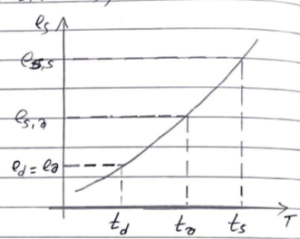Monday, October 2, 2023

# Penmans Equation | Engineering Hydrology | Calculation of Potential Evapotranspiration by Penmans Equation

### 1. Introduction

Penman’s equation is the semi-empirical equation used for the calculation of potential Evapotranspiration using various meteorological data. The daily potential evapotranspiration.

Potential Evapotranspiration (PET) = AxHo+ γ Ea / (A+ γ)

### 2. Derivation of Penmans Equation

As we know,

Hn = G+E   ——-(i)

where,

Hn= net solar radiation with a unit of mm of evaporable water per day.

G= energy used for heating for air

E= energy used for evapotranspiration

Now, from Dalton’s law

G = γ f(v) (ts-ta)

where,

γ= Psychrometric constant = 0.49 mmhg/ºC

f(v)= Function of wind speed

ta = temperature of air

t= dew point

ts = temperature of water surfaceThen,

G = γ f(v) {(ts-td)- (ta-td)}

G = γ f(v) {(es,s -ed)/A- (ea-ed)/A}

G = γ f(v) {(es,s -ed)- (ea-ed)}/A

Where, es = saturated vapour pressure for ts.

eo=ed= actual vapour pressure

es= Saturated vapour pressure for ta.

A = Slope of saturation vapour pressure curve.

then, G= γ{E-Ea }/ A

Where, Ea = acrodynamic evapouration

then, from eqn i and ii, we get

Hn = G+E

Hn= γ{E-Ea }/ A + E

E = ( HA + γE)/ (A+γ)

Which is a required expression for penmans equation.

 Read Also: Derivation of Unit HydrographHe is a founder and lead author of Dream Civil International and his civil engineering research articles has been taken as source by world's top news and educational sites like USA Today, Time, The richest, Wikipedia, etc.
Latest Articles

Related Articles